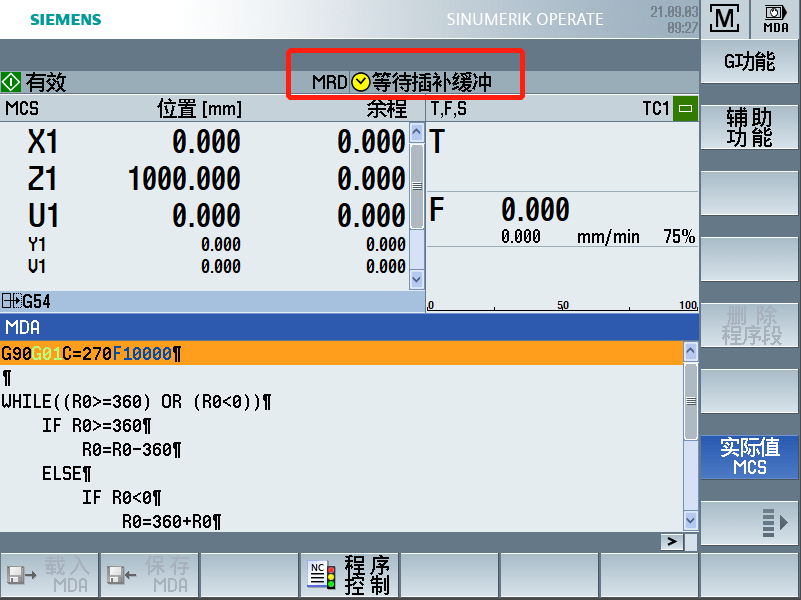``````WHILE((R0>=360) OR (R0<0))
IF R0>=360
R0=R0-360
ELSE
IF R0<0
R0=360+R0
ENDIF
ENDIF
ENDWHILE``````